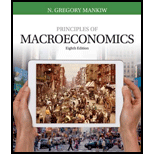Chapter 4, Problem 8PA### Principles of Macroeconomics (Mind...

8th Edition
N. Gregory Mankiw
ISBN: 9781305971509

#### Solutions

Chapter
Section### Principles of Macroeconomics (Mind...

8th Edition
N. Gregory Mankiw
ISBN: 9781305971509
Textbook Problem

# The market for pizza has the following demand and supply schedules: Price Quantity Demanded Quantity Supplied \$4 135 pizzas 26 pizzas 5 104 53 6 81 81 7 68 98 8 53 110 9 39 121 a. Graph the demand and supply curves. What are the equilibrium price and quantity in this market? b. If the actual price in this market were above the equilibrium price, what would drive the market toward the equilibrium? c. If the actual price in this market were below the equilibrium price, what would drive the market toward the equilibrium? d. e.

Subpart (a):

To determine
Demand and supply and its equilibrium.

Explanation

Figure 1 illustrates the equilibrium condition in the economy.

Figure 1 illustrates the market for pizzas...

Subpart (b):

To determine
Demand and supply and its equilibrium.

Subpart (c):

To determine
Demand and supply and its equilibrium.

### Still sussing out bartleby?

Check out a sample textbook solution.

See a sample solution

#### The Solution to Your Study Problems

Bartleby provides explanations to thousands of textbook problems written by our experts, many with advanced degrees!

Get Started